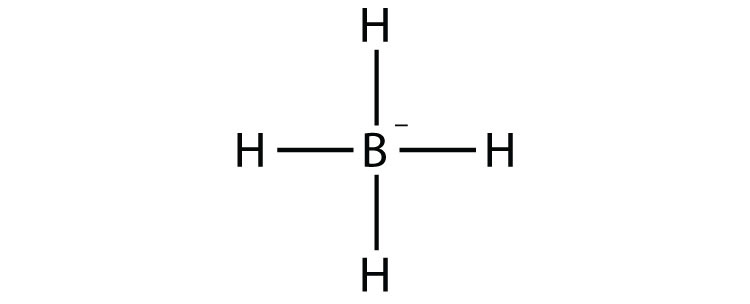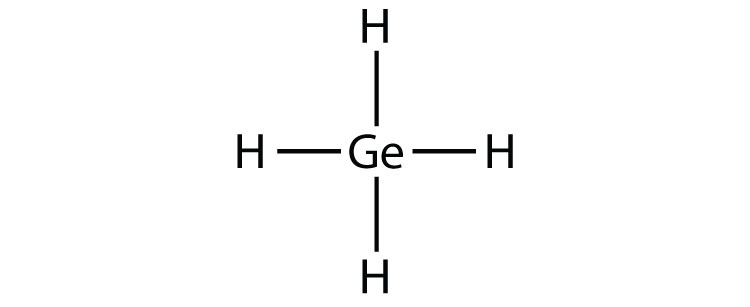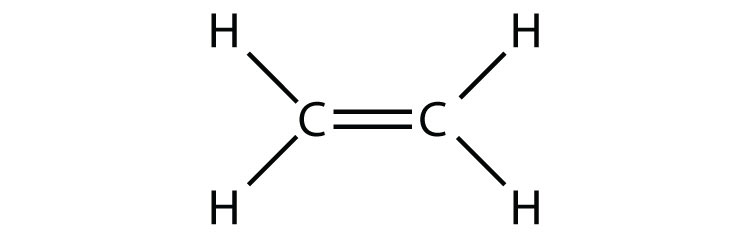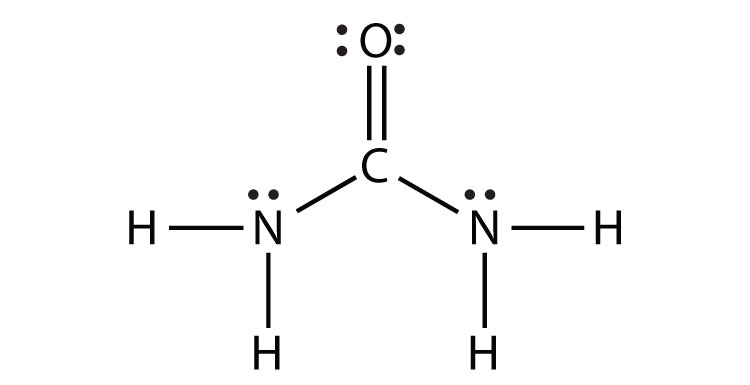QUESTION ANSWER 1. How many electrons will be in the valence shell of H atoms when it makes a covalent bond? 1. two 3. What is the Lewis electron dot diagram of I2? Circle the electrons around each atom to verify that each valence shell is filled. 3.5. What is the Lewis electron dot diagram of NCl3? Circle the electrons around each atom to verify that each valence shell is filled. 5.7. Draw the Lewis electron dot diagram for each substance. SF2 BH4− 7.9. Draw the Lewis electron dot diagram for each substance. GeH4 ClF 9.11. Draw the Lewis electron dot diagram for each substance. Double or triple bonds may be needed. SiO2 C2H4 (assume two central atoms) 11.13. Draw the Lewis electron dot diagram for each substance. Double or triple bonds may be needed. CS2 NH2CONH2 (assume that the N and C atoms are the central atoms) 13.